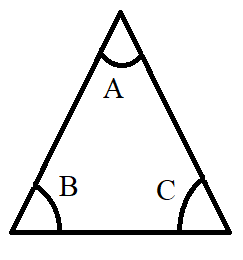Courses
Courses for Kids
Free study material
Offline Centres
MoreLast updated date: 09th Dec 2023
Total views: 281.4k
Views today: 8.81k

# If A, B and C are the interior angles of a triangle, then show that: $\sin \left( {\dfrac{{B + C}}{2}} \right) = \cos \left( {\dfrac{A}{2}} \right)$Verified
281.4k+ views
Hint: The given question deals with basic simplification of trigonometric functions by using some of the simple trigonometric formulae such as $\sin \left( {{{90}^ \circ } - \theta } \right) = \cos \theta$. Basic algebraic rules and trigonometric identities are to be kept in mind while doing simplification in the given problem and proving the result given to us.

Complete step-by-step solution:
In the given problem, we have to prove a trigonometric equality that can be further used in many questions and problems as a direct result and has wide ranging applications. For proving the desired result, we need to have a good grip over the basic trigonometric formulae and identities.
We are given that the angles A, B and C are the interior angles of a triangle.Now, using the angle sum property of the triangle, we know that the sum of all the three interior angles of the triangle is ${180^ \circ }$. So, we get, $A + B + C = {180^ \circ }$.
So, we have, $A + B + C = {180^ \circ }$
Shifting the angle A from left side of the equation to right side of equation, we get,
$\Rightarrow B + C = {180^ \circ } - A$
Now, dividing both sides of the equation by $2$, we get,
$\Rightarrow \left( {\dfrac{{B + C}}{2}} \right) = \left( {\dfrac{{{{180}^ \circ } - A}}{2}} \right)$
Now, taking sine on both sides of the equation, we get,
$\Rightarrow \sin \left( {\dfrac{{B + C}}{2}} \right) = \sin \left( {\dfrac{{{{180}^ \circ } - A}}{2}} \right)$
Simplifying the expression, we get,
$\Rightarrow \sin \left( {\dfrac{{B + C}}{2}} \right) = \sin \left( {{{90}^ \circ } - \dfrac{A}{2}} \right)$
Now, we know that sine and cosine are the complementary trigonometric ratios. So, we have, $\sin \left( {{{90}^ \circ } - \theta } \right) = \cos \theta$.
$\Rightarrow \sin \left( {\dfrac{{B + C}}{2}} \right) = \cos \left( {\dfrac{A}{2}} \right)$
So, we arrive at the equation that we needed to prove in the problem given to us.
Hence, Proved.

Note: Given problem deals with Trigonometric functions. For solving such problems, trigonometric formulae should be remembered by heart. Besides these simple trigonometric formulae, trigonometric identities are also of significant use in such types of questions where we have to simplify trigonometric expressions with help of basic knowledge of algebraic rules and operations.